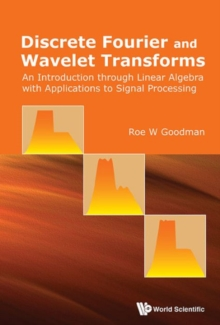# Discrete Fourier And Wavelet Transforms: An Introduction Through Linear Algebra With Applications To Signal Processing Paperback / softback

#### Description

This textbook for undergraduate mathematics, science, and engineering students introduces the theory and applications of discrete Fourier and wavelet transforms using elementary linear algebra, without assuming prior knowledge of signal processing or advanced analysis.It explains how to use the Fourier matrix to extract frequency information from a digital signal and how to use circulant matrices to emphasize selected frequency ranges.

It introduces discrete wavelet transforms for digital signals through the lifting method and illustrates through examples and computer explorations how these transforms are used in signal and image processing.

Then the general theory of discrete wavelet transforms is developed via the matrix algebra of two-channel filter banks.

Finally, wavelet transforms for analog signals are constructed based on filter bank results already presented, and the mathematical framework of multiresolution analysis is examined.

#### Information

• Format: Paperback / softback
• Pages: 300 pages
• Publisher: World Scientific Publishing Co Pte Ltd
• Publication Date:
• Category: Numerical analysis
• ISBN: 9789814725774

£40.00

£31.68

Free delivery within the UK